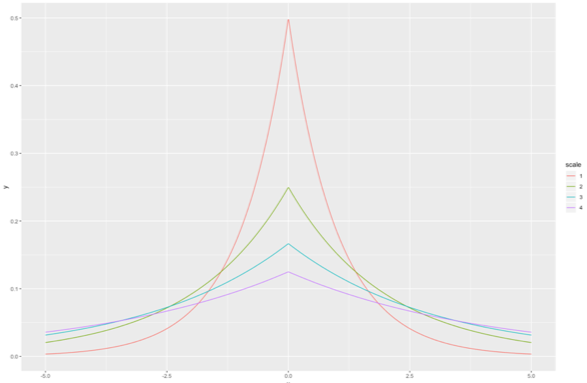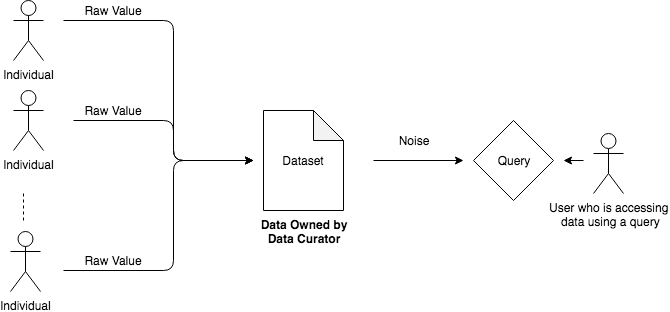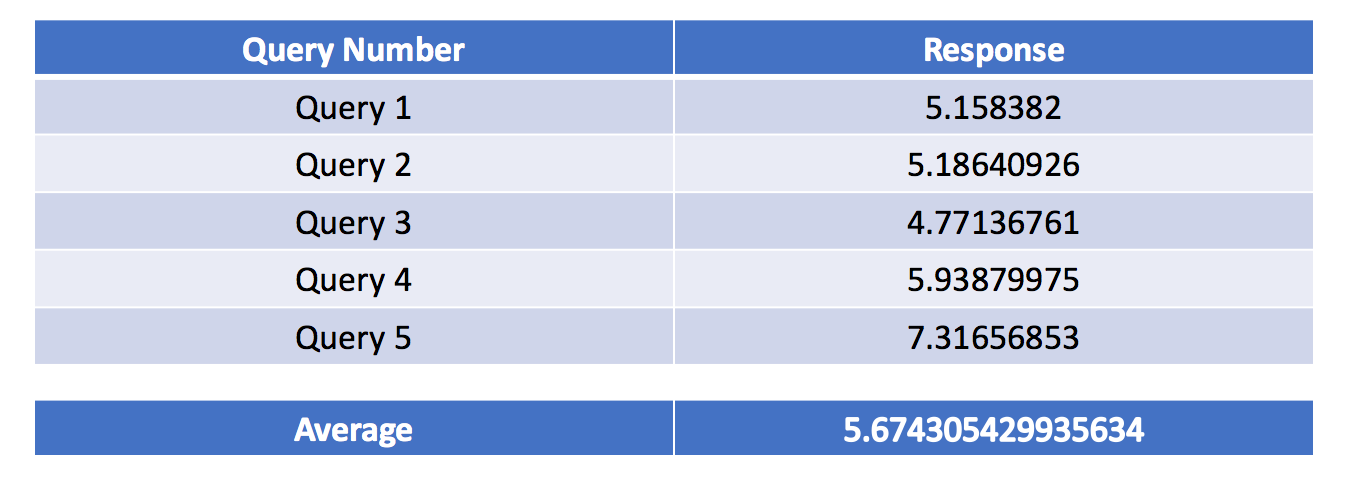# Protect User Data with Differential Privacy

Researcher at CVC, UAB BarcelonaIn 2018, Cambridge Analytica had accessed personal information of over 50 million Facebook users and used this to influence election results. With such data breaches happening all over the world , users are concerned about how companies handle their private information now more than ever.

Amidst such rising concerns, developers are trying to implement techniques which protect user privacy right from the beginning, i.e. at the software level.

Could removing the personal identifiers from a dataset help?

Anonymizing a dataset -- that is, stripping the dataset of private details like name and address -- may seem like the go-to option to protect user data. But, as shown in studies, information from such an anonymized dataset can be re-identified. For example, the anonymized health records of the governor of Massachusetts, William Held, were re-identified using publically available voting records. 

Differential Privacy

A technique introduced by Cinthia Dwork , differential privacy (DP) protects user privacy by adding random noise to the data. For example, adding three years to your age while answering a survey protects your information. As the data provided is noisy, it cannot be used to identify a user.Differential Privacy Mechanism

But how would one get data while protecting user privacy?

The ingenuity of DP is that it protects user privacy while allowing meaningful analysis to happen over the dataset. By adding noise to individual data points, it protects the user’s privacy, but on aggregating these data points, the noise is averaged out, obtaining a result closer to the original one.

Consider that users are asked, “Have you ever broken the law?”.

To save themselves from the implications of answering honestly, the users who would have broken the law would also end up responding 'no' to this question, resulting in a dataset which is of no use to a data analyst.

To prevent users from answering 'no' to this question irrespective of their honest answer, the data curator allows them to respond following a randomized procedure.

1. If heads comes on tossing a coin, then users have to answer honestly.
2. If tails comes on tossing a coin, then users toss another coin and answer based on that, i.e. yes for heads on a second coin flip and no for tails.

This procedure provides ‘plausible deniability’ to the respondents. By basing the answers on random coin flips, one cannot know whether the data owner provided accurate data or not, in turn protecting user privacy.

If the true results would have been:

1. true_db = [1,1,0,0,1]
2. first coin flip = [1,0,0,1,0]
3. second Coin Flip = [0,0,1,1,1]

Then after following randomized response process:

augmented_db = [1,0,1,0,1], where 1 stands for yes and 0 for no.Randomized Response Procedure

To generate an augmented database using code:Randomized Response

But wouldn’t the patterns formed from analyzing this noisy data be useless?

Let’s analyze the data collected using the randomized response procedure.

The probability that a person has committed a crime = (no. of people who have committed a crime/total no. of people)

To know the actual probability, the data analyst can then de-skew this result using basic mathematics as illustrated below.

Following,Equation 1: Generate an augmented Database using randomized response

we observe,Equation 2: Probability of an event over the augmented database

Let's assign values to these probabilities.

P(augmented db = 1) = 0.6 and P(true db = 1) = p (where let’s take the value of p to be 0.7. The analyst doesn’t know this yet). Since we are using unbiased coins, P(first coin flip = 1) = 0.5 and P(second coin flip = 1) = 0.5. Then, using equation 2,

=> 0.6 = 0.5 * p + 0.5* 0.5
=> 0.6 = 0.5 * (p + 0.5)
=> 0.6 / 0.5 = (p + 0.5)
=> 2*0.6 = p + 0.5
=> 1.2–0.5 = p
=> p = 0.7, the probability that a person has committed a crime as per true_db

This is also applicable if let’s say the first coin used is biased with a probability of P(first_coin_flip = 1) = noise.

Using the second equation again,=> P(augmented_db) = P(true_db) * noise + (1-noise)*0.5
=> P(true_db) * noise = P(augmented_db) — (1-noise)*0.5
=> P(true_db) = (P(augmented_db) — (1-noise)*0.5) / noise 

The correct result is achievable only in extensive databases where the noise filters out. In reality, the result obtained would be close to the correct value.Analysis using the augmented database

DP requires the output of a query to be the same when a user is removed from the original dataset. Since the query result doesn’t vary, the privacy of that user is protected. By definition, this property becomes a property of the query and should be applicable over all databases.

Being too strong a requirement for a query, DP instead quantifies the information leaked about a database over a query.

In the previous example, noise is added using a randomized response, but in real-life cases, noise is added by taking numbers from Laplacian or Gaussian distribution with a variance ofLaplace distribution plots

Here the epsilon (e) parameter is the privacy loss quantified by DP, making a query epsilon-differentially private. . Noise added to the dataset increases as this epsilon value decreases, resulting in better privacy guarantees but leading to less accurate results.

According to experts, epsilon values between 0 to 1 are very good, 1 to 10 are okay, with privacy protection degrading as epsilon increases more than 10. 

Types of Differential Privacy Techniques

Local Differential Privacy:

In this case, noise is added directly to the data by the individual data owners – for example, adding three years to your age or subtracting five thousand from your salary while replying to a survey. Doing this, the data curator, the person who is aggregating the dataset, doesn’t know the actual value, and thus privacy is protected. In this case, more noise gets added in total and to arrive leading to the requirement of an extensive database to do accurate analysis on the database.Local Differential Privacy

Global Differential Privacy:

In this case, noise is added by the data curator to the output of a query on the database -- for example, adding 200 to the number of people with a salary higher than 10,000 after calculating the correct figure from the curated database. Doing this, the data curator protects user privacy from people who are querying the database. In this case, less noise gets added as compared to local DP, and one can obtain accurate analysis even with a smaller database.Global Differential Privacy

Limitations of Differential Privacy

Can one not just average out the noise for malicious purposes?

Consider the following responses to a query on a database after adding noise:Queries answers generated for true value of 5.5

The data behind the queries is 5.5, and the arrived outcome is 5.67.

As shown, by averaging out these query responses, one can arrive at a result close to the exact one. Estimating sensitive values from the database by repeatedly querying the database is a significant limitation of differential privacy.

Privacy Budget

As shown in the previous example, the aggregation of queries leads to a higher privacy loss. One can overcome this limitation by defining a privacy budget.

Privacy budget essentially means an upper threshold for total privacy loss. One stops answering queries when the loss increases more than the allocated budget.

Machine Learning and Privacy

In the domain of machine learning, algorithmic models are trained over a database to learn general patterns about the database. While learning these patterns, there is a high chance that these model also learn private details about the data itself.

A malicious user can interpret these private details by analyzing the parameters of such a trained model. Thus, it becomes imperative to include privacy protecting techniques such as DP while training machine learning models. A training epoch over the database is like a query to the database. A model is trained as long as the privacy loss is within the privacy loss budget.

Abadi et al. recently proposed a new variant of the optimization algorithm, private SGD (a privacy-preserving stochastic gradient descent), to train deep neural networks. 

Applications

Google, an early proponent of such techniques, has used DP to collect usage data about Chrome and which websites were majorly crashing in a privacy-preserving way.  Apple uses DP to gather information about emojis used in IoS devices. 

Misuses

While companies have been adopting DP, there is a debate whether they are doing it correctly or not. Technically, the privacy budget should be permanent, and a user should get blocked from querying once the loss exceeds the privacy budget. But, as described by researchers , Apple refreshes the privacy budget every new day, giving them the ability to exhaust their budget in a day and then start with a new budget the next day. 

Conclusive Thoughts

Differential privacy is a promising privacy-protecting technique, as it overcomes the limitations of earlier methods. It also provides an explicit privacy guarantee.

Though companies are not very clear about the implementation details at present, they are coming up with new applications which follow privacy-preserving techniques.

While DP parameters, such as epsilon, have statistical meaning, there is no recipe as for now for choosing values for these parameters. A lot of research is happening in this area, and this area looks very promising. With new courses coming up to teach Differential Privacy concepts , DP indeed will become an essential part of software applications.

Refer the entire code here:

https://gist.github.com/Tandon-A/c5a2fafb15982ed5c1d543ba9331d57bThis post was previously published on Medium

References

1. Data Breach Wikipedia Article [https://en.wikipedia.org/wiki/Data_breach]
2. The “Re-identification” of Governor William Weld’s Medical Information [https://fpf.org/wp-content/uploads/The-Re-identification-of-Governor-Welds-Medical-Information-Daniel-Barth-Jones.pdf]
3. Differential Privacy: A survey of results [https://web.cs.ucdavis.edu/~franklin/ecs289/2010/dwork_2008.pdf]
4. Deep Learning with Differential Privacy [https://arxiv.org/pdf/1607.00133.pdf]
5. UN Handbook on Privacy-Preserving Computation Techniques [http://publications.officialstatistics.org/handbooks/privacy-preserving-techniques-handbook/UN%20Handbook%20for%20Privacy-Preserving%20Techniques.pdf]
6. Differential Privacy Overview [https://www.apple.com/privacy/docs/Differential_Privacy_Overview.pdf]
7. Understanding differential privacy and why it matters for digital rights [https://www.accessnow.org/understanding-differential-privacy-matters-digital-rights/]
8. Privacy Loss in Apple’s Implementation of Differential Privacy on MacOS 10.12 [https://arxiv.org/pdf/1709.02753.pdf]
9. A brief introduction to differential privacy [https://medium.com/georgian-impact-blog/a-brief-introduction-to-differential-privacy-eacf8722283b]
10. Differential Privacy: Secure and Private AI course on Udacity [https://www.udacity.com/course/secure-and-private-ai--ud185]